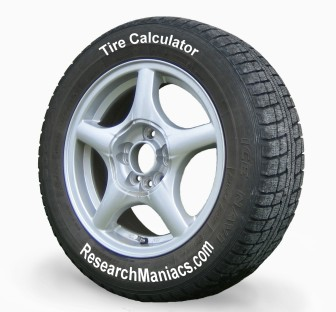Calculate Tire Diameter How do you calculate Tire Diameter

So how do you calculate the diameter of your tire based on the letters and numbers printed on it?

Basically all tires have something like this printed on it:
P315/70R17

And if we label all the number above like this:
Type Width / Ratio Radial Rim

Then the tire diameter formula is as follows:
(((Width x (Ratio/100)) x 2)/ 25.4) + Rim = Tire Diameter

However, if you don't have a calculator handy, you can simply fill in the information here and we will calculate the tire diameter for you:

 Tire Diameter Calculator WIDTH RATIO RIM ANSWER / R = Calculator by ResearchManiacs.com
Here is a more detailed explanation of the different parts printed on the tire:

Type: Tells you what kind of tire it is:

P = Passenger vehicles tire (most cars and trucks)
T = Temporary tire (smaller tire)
LT = Light Truck tire (larger SUVs, pickups etc)
ST = Special Trailer (boat trailer etc.)

Width: This number tells you the width in millimeter of the inner walls of the tire. (you can devide millimeter by 25.4 to get inches)

Ratio: This number tells you the distance from rim to tread as a percentage of the width above.

R = Radial (you can sometimes also find D for Diagonal and B for belted)

Rim: The last number indicates the inner diameter and should match the wheel and range from 9 to 28.